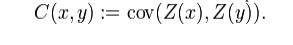Home | | Communication Theory | Important Short Questions and Answers: Communication Theory - Random Process

# Important Short Questions and Answers: Communication Theory - Random Process

Communication Theory - Random Process - Important Short Questions and Answers: Communication Theory - Random Process

RANDOM PROCESS

1. Define random variables.

A random variable, usually written X, is a variable whose possible values are numerical outcomes of a random phenomenon. Random variable consists of two types they are discrete and continuous type variables.

2. What is meant by probability distribution?

The probability distribution of a discrete random variable is a list of probabilities associated with each of its possible values. It is also sometimes called the probability function or the probability mass function.

3. What are the conditions applied in the central limit theorem?

 The mean of the population of means is always equal to the mean of the parent population from which the population samples were drawn.

 The standard deviation of the population of means is always equal to the standard deviation of the parent population divided by the square root of the sample size (N).

 The distribution of means will increasingly approximate a normal distribution as the size N of samples increases.

4.  Define stationary process.

Stationary process is a stochastic process whose joint probability distribution does not change when shifted in time. Consequently, parameters such as the mean and variance, if they are present, also do not change over time and do not follow any trends.

5.Write the equation for correlation?

The population correlation coefficient ρX,Y between two random variables X and Y with expected values μX and μY and standard deviations σX and σY is defined as:6. what is meant by covariance?

Covariance is a measure of how much two variables change together, and the covariance function, or kernel, describes the spatial covariance of a random variable process or field.7. Define random process.

A random process X(t) is a Gaussian process if for all n and all (t1 ,t2 ,…,tn ), the random variables have a jointly Gaussian density function.

8. Write the equation of Autocorrelation?

The autocorrelation function of the output random process Y (t). By definition, we have

RY (t, u) = E[Y (t)Y (u)]

where t and u denote the time instants at which the process is observe

9.Write the applications of random process?

The available noise power is directly proportional to temperature and it is independent of value of resistance. This power specified in terms of temperature is called as noise temperature. It is denoted by Te . It is given as,

•   Te =(F−1)T A Gaussian process can be used as a prior probability distribution over functions in Bayesian inference.

• Wiener process (aka Brownian motion) is the integral of a white noise Gaussian process. It is not stationary, but it has stationary increments.

Study Material, Lecturing Notes, Assignment, Reference, Wiki description explanation, brief detail
Communication Theory : Random Process : Important Short Questions and Answers: Communication Theory - Random Process |